+
Solve Linear Inequalities
Solving Linear Equations and Inequalities
0
of 0 possible points

# Solve Linear Inequalities

Author: Sophia Tutorial
##### Description:

Solve a linear inequality.

(more)

Sophia’s self-paced online courses are a great way to save time and money as you earn credits eligible for transfer to many different colleges and universities.*

No credit card required

37 Sophia partners guarantee credit transfer.

299 Institutions have accepted or given pre-approval for credit transfer.

* The American Council on Education's College Credit Recommendation Service (ACE Credit®) has evaluated and recommended college credit for 33 of Sophia’s online courses. Many different colleges and universities consider ACE CREDIT recommendations in determining the applicability to their course and degree programs.

Tutorial
what's covered
1. Graphing Inequalities
2. Solving Linear Inequalities

# 1. Graphing Inequalities

Solving inequalities is very similar to solving equations with one exception. if we multiply or divide by a negative number, the symbol will need to flip directions. We will keep that in mind as we solve inequalities.

hint
When multiplying or dividing by a negative number, the inequality sign switches. For example, greater than becomes less than, and less than becomes greater than.

# 2. Solving Linear Inequalities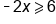Divide both sides by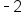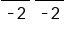Divide by a negative - flip symbol!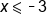Graph, starting at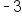, going down with ] for less than or equal to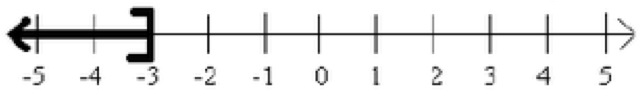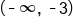Interval Notation

The inequality we solve can get as complex as the linear equations we solved. We will use all the same patterns to solve these inequalities as we did for solving equations. Just remember that any time we multiply or divide by a negative the symbol switches directions (multiplying or dividing by a positive does not change the symbol!)

 Solve and give Interval notation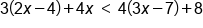Distribute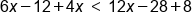Combine like terms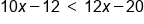Move variable to one side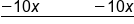Subtract 10x from both sides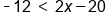Add 20 to both sides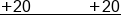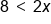Divide both sides by 2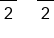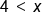Be careful with graph, x is larger!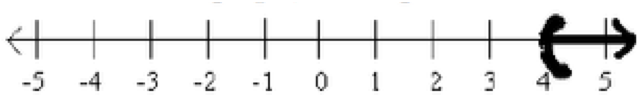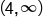Interval Notation

summary
When graphing inequalities, it is important to be careful when the inequality is written backwards as in the above example (4 less than x rather than x greater than 4). Often students draw their graphs the wrong way when this is the case. The inequality symbol opens to the variable, this means the variable is greater than 4. So we must shade above the 4.
Solving linear inequalities is similar to solving equations, except that we use an inequality symbol instead of an equal sign. When we're solving an inequality and you multiply or divide by a negative number, your inequality symbol is going to switch directions. Also, when we're solving a compound inequality, any operation done between the inequality symbols must be done on the other side of both inequality symbols.

Source: Adapted from "Beginning and Intermediate Algebra" by Tyler Wallace, an open source textbook available at: http://wallace.ccfaculty.org/book/book.html

Rating